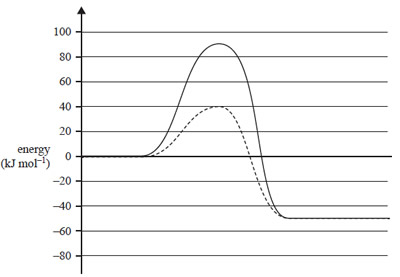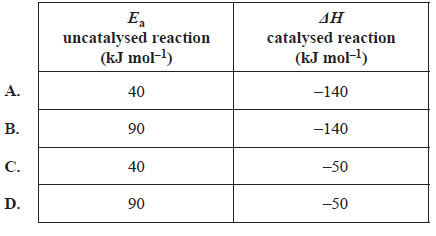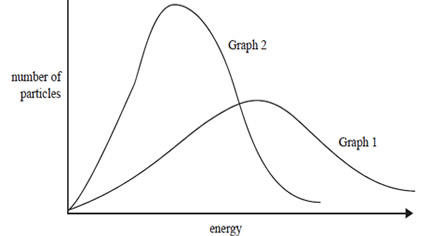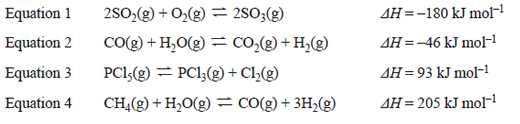Thermochemistry (2018 VCE) 1) The energy profile diagram below represents a particular reaction. One graph represents the uncatalysed reaction and the other graph represents the catalysed reaction .Which of the following best matches the energy profile diagram?Solution 2) An equation for the complete combustion of methanol is 2CH3OH(l) + 3O2(g) → 2CO2(g) + 4H2O(g) ΔH for this equation would be A. +726 kJ mol–1 B. –726 kJ mol–1 C. +1452 kJ mol–1 D. –1452 kJ mol–1. Solution 3) The kinetic energy of a sample of gas in a container of fixed volume is represented by the distribution curve shown in Graph 1 below. One change was made to the sample and the resulting distribution curve of kinetic energy is shown in Graph 2.Which one of the following statements explains the change from Graph 1 to Graph 2? A. The average kinetic energy of the gas molecules decreased. B. More gas, at the same temperature, was added to the container. C. More collisions occurred between gas particles. D. The temperature of the gas was increased. Solution4) The four equations below represent different equilibrium systems.After equilibrium was established in each system, the temperature was decreased and the pressure was increased. In which equilibrium system would both changes result in an increase in yield? A. Equation 1 B. Equation 2 C. Equation 3 D. Equation 4 Solution 5) The molar heat of combustion of pentan-1-ol, C5H11OH, is 3329 kJ mol–1. M(C5H11OH) = 88.0 g mol–1 The mass of C5H11OH, in tonnes, required to produce 10 800 MJ of energy is closest to A. 0.0286 B. 0.286 C. 2.86 D. 286 Solution6) Hydrogen peroxide, H2O2, in aqueous solution at room temperature decomposes slowly and irreversibly to form water, H2O, and oxygen, O2, according to the following equation. 2H2O2(aq) → 2H2O(l) + O2(g) ΔH < 0 a. What effect will increasing the temperature have on the rate of O2 production? Use collision theory to explain your answer. Solutionb) When a small lump of manganese(IV) dioxide, MnO2, is added to the H2O2 solution, the rate of O2 production increases, but when powdered MnO2 is added instead, the rate of O2 production is greatly increased. The MnO2 is recovered at the end of the reaction. State the function of MnO2 in this reaction. Solution c) A solution of H2O2is labelled '10 volume' because 1.00 L of this solution produces 10.0 L of O2 measured at standard laboratory conditions (SLC) when the H2O2 in the solution is fully decomposed. Calculate the concentration of H2O2 in the '10 volume' solution, in grams per litre, when this solution is first prepared. Solution d) Propose a method to determine how quickly a solution of H2O2 decomposes when stored at a particular temperature. Solution